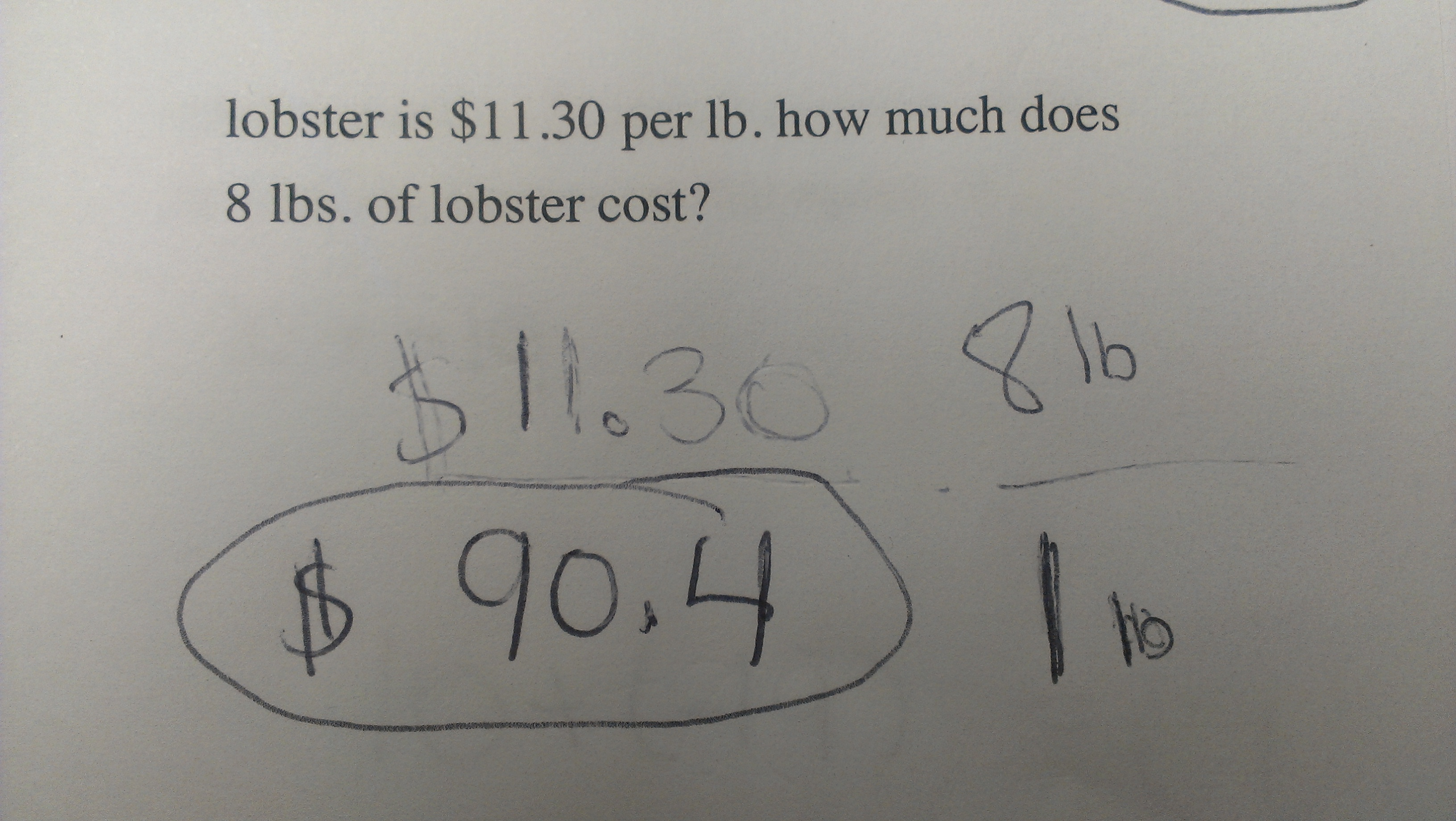Categories

# MoneyLet’s not even call this a mistake quite yet. What does this answer reveal about what the kid knows?

## 3 replies on “Money”

tieandjeanssays:

Using the hyper zoom, I don’t see an equals sign. That’s tenuous, I know, but it means that I’m likely to read the work as the student sequentially recording problem info from left to right (11.30 … 8lb) then realizing that 11.30 is really the 1lb price, multiplying and writing their answer in the space left over.

I’ not sure if I’d count leaving off the cents zero when they copied the answer from a calculator as an error.

Rachelsays:

Kid knows how much 8 lbs of lobster cost! And knows that there is a relationship between the unit cost & total cost, as well as the unit weight & total weight.
…just needs to clarify that he’s making the correct comparisons between all those values.

Jaspersays:

The student has clearly circled \$ 90.4 as the answer, which is correct.

The student’s work is a bit unclear. It might mean:

(price) * (quantity) = ((\$ 11.30) / (1 lb)) * (8 lb) = \$ 11.30 * 8 = \$ 90.4

or it might mean:

(price) * (ratio) = \$ 11.30 * (8 lb / 1 lb) = \$ 11.30 * 8 = \$ 90.4

Both approaches are correct; I prefer the first one.

It would be easier to understand if the student clearly had the answer on a separate line, as opposed to sharing a line used for an intermediate step.